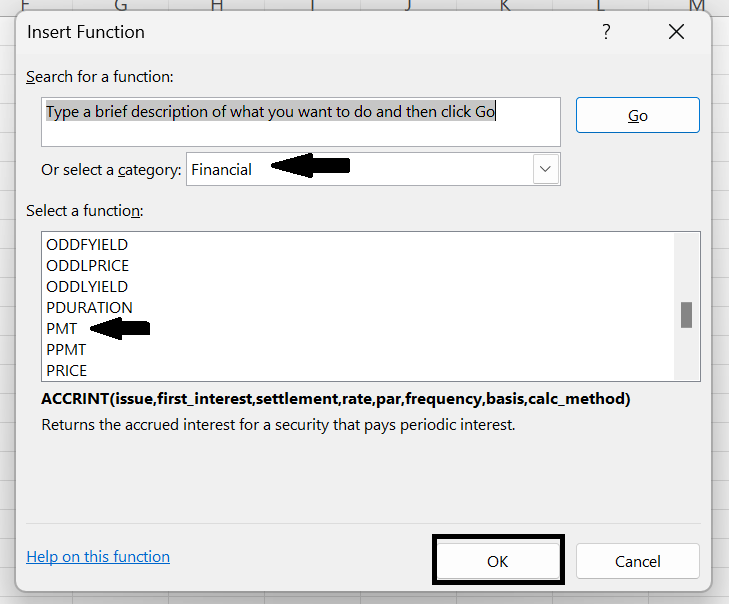top of page
Search

# How to use PMT Function in Excel

The PMT function in Excel is used to calculate the periodic payment for a loan or investment based on a constant interest rate, the number of payments, and the present value of the loan or investment.

The syntax for the PMT function is as follows:

`=PMT(rate, nper, pv, [fv], [type])`

where:

• rate: The interest rate per period.

• nper: The total number of payment periods.

• pv: The present value of the loan or investment.

• [fv]: Optional. The future value of the loan or investment. If omitted, it is assumed to be 0.

• [type]: Optional. The timing of the payment. If 0 or omitted, payments are assumed to be due at the end of the period. If 1, payments are assumed to be due at the beginning of the period.

For example, to calculate the monthly payment for a \$10,000 loan with a 5% annual interest rate over a 5-year term, you can use the following formula:

`=PMT(5%/12, 5*12, 10000)`

This will return a result of -\$188.71, indicating that the monthly payment required to pay off the loan in 5 years is \$188.71. The negative sign indicates that the payment is an outgoing cash flow.

## How to use the PMT Function in Excel

There are three different methods to use the PMT function in Excel:

1. Using basic formula within the cell

2. Using Insert Function.

3. Using Formula Tab

### Method 1: Using Basic formula within the cell

STEP 1: Open Microsoft Excel.

STEP 2: Consider the table below where we have mentioned the amount of loan, Interest rate and total number of years for which you want the loan.

STEP 3: Select the cell where you want to display the result.STEP 4: Now, after selecting the cell type "=PMT( )" then bracket.

STEP 5: Inside the bracket,

• Type B3/12. B3 contains the interest rate. It is divided by 12 to convert a yearly interest rate to a monthly interest rate. Then place a comma.

• Type B4 * 12. B4 contains the year which will contribute to the total payments for the loan, but because we are paying monthly payments, we have to multiply the number of years by twelfth to the number of payments. Then Comma.

• Type B2. It contains the loan amount.

• Close the bracket.STEP 6: Press Enter. You will get your result.Note: The Excel PMT function returns a negative value because this represents payments being made from you to your lender. Alternatively, if you prefer the PMT function to return a positive value you can enter the Loan Amount as a negative figure

### Method 2: Using Insert Function

STEP 1: Click on the Insert Function option located above the spreadsheet.STEP 2: A dialogue box will appear. Select the "Financial" category.

STEP 3: Select "PMT" from the Select a Function group and click OK.STEP 4: A Function Arguments dialogue box will appear.

1. In "Rate", type B3/12.

2. In "Nper, type B4*12"

3. In "Pv", type B2STEP 5: Click OK.

### Method 3: Using Formula Tab

STEP 1: Click on the Formula Tab and select the "Financial" option.

STEP 2: Select the "PMT" option.STEP 3: An Arguments Function dialogue box will appear.

Enter the necessary details and then click OK.STEP 4: You will get your result.#### Benefits:

1. Quick and easy calculation of fixed periodic payments for loans or investments.

2. Flexibility to work with various types of loans or investments.

3. Ability to compare different loan or investment options and analyze the impact of different variables on the total cost or return.

#### Limitations:

1. Assumes a fixed interest rate, which may not be accurate for loans or investments with variable rates.

2. Does not account for other fees or charges associated with loans or investments.

3. Does not account for changes in interest rate or payment amounts over time, which may reduce accuracy for long-term analysis.

4. May not be suitable for complex financial calculations or for loans or investments with non-standard terms.

### Conclusion

The PMT function can be used for various types of loans or investments, but it is designed for those with fixed interest rates. You can use the PMT function to compare different loans or investments and analyze the impact of different variables on the total cost or return of the loan or investment.

#### Question 1: What is the result of the PMT function?

Answer: The result of the PMT function is the fixed periodic payment required to pay off the loan or investment over the specified period of time.

#### Question 2: How do I use the PMT function in Excel?

Answer: To use the PMT function, select a cell where you want to display the result, type "=PMT(" and then input the arguments in the correct order, separated by commas. Press Enter to display the result.

#### Question 3: Can the PMT function be used for different types of loans or investments?

Answer: Yes, the PMT function can be used for different types of loans or investments, including mortgages, car loans, personal loans, and investments with fixed returns.

#### Question 4: Can the PMT function be used for loans or investments with variable interest rates?

Answer: No, the PMT function is designed for loans or investments with fixed interest rates. For loans or investments with variable interest rates, you would need to use a different formula or function to calculate the periodic payment.

#### Question 5: How can I use the PMT function to compare different loans or investments?

Answer: You can use the PMT function to calculate the periodic payment for different loans or investments and compare them to see which one is more affordable or profitable. You can also use the PMT function in conjunction with other Excel functions to analyze the impact of different interest rates, payment periods, or loan amounts on the total cost or return of the loan or investment.